Next: Conditional Variation Up: Classical Mechanics Previous: Generalized Momenta

# Calculus of Variations

It is a well-known fact, first enunciated by Archimedes, that the shortest distance between two points in a plane is a straight-line. However, suppose that we wish to demonstrate this result from first principles. Let us consider the length,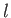, of various curves,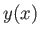, that run between two fixed points,and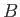, in a plane. Now,takes the form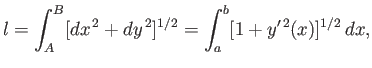(B.29)

where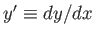. Note thatis a function of the function. In mathematics, a function of a function is termed a functional.

In order to find the shortest path between pointsand, we need to minimize the functionalwith respect to small variations in the function, subject to the constraint that the end points,and, remain fixed. In other words, we need to solve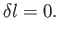(B.30)

The meaning of the previous equation is that if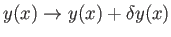, where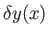is small, then the first-order variation in, denoted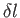, vanishes. In other words,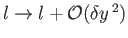. The particular functionfor which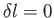obviously yields an extremum of(i.e., either a maximum or a minimum). Hopefully, in the case under consideration, it yields a minimum of.

Consider a general functional of the form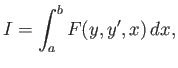(B.31)

where the end points of the integration are fixed. Suppose that. The first-order variation in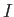is written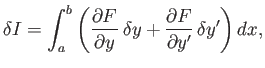(B.32)

where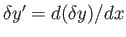. Setting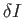to zero, we obtain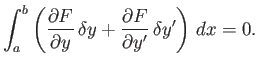(B.33)

This equation must be satisfied for all possible small perturbations,.

Integrating the second term in the integrand of the previous equation by parts, we get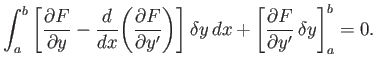(B.34)

If the end points are fixed then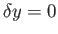at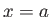and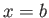. Hence, the last term on the left-hand side of the previous equation is zero. Thus, we obtain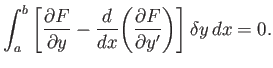(B.35)

The previous equation must be satisfied for all small perturbations. The only way in which this is possible is for the expression enclosed in square brackets in the integral to be zero. Hence, the functionalattains an extremum value whenever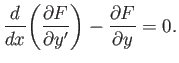(B.36)

This condition is known as the Euler-Lagrange equation.

Let us consider some special cases. Suppose that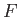does not explicitly depend on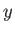. It follows that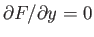. Hence, the Euler-Lagrange equation, (B.36), simplifies to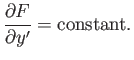(B.37)

Next, suppose thatdoes not depend explicitly on. Multiplying Equation (B.36) by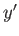, we obtain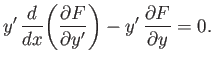(B.38)

However,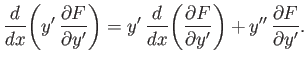(B.39)

Thus, we get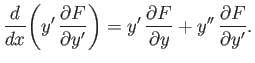(B.40)

Ifis not an explicit function ofthen the right-hand side of the previous equation is the total derivative of, namely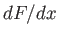. Hence, we obtain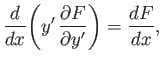(B.41)

which yields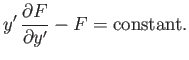(B.42)

Returning to the case under consideration, we have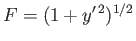, according to Equations (B.29) and (B.31). Hence,is not an explicit function of, so Equation (B.37) yields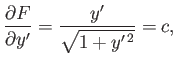(B.43)

where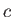is a constant. So,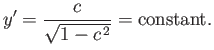(B.44)

Of course,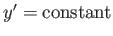is the equation of a straight-line. Thus, the shortest distance between two fixed points in a plane is indeed a straight-line.Next: Conditional Variation Up: Classical Mechanics Previous: Generalized Momenta
Richard Fitzpatrick 2016-01-25